# Case Sensitive Lookup – Excel & Google Sheets

## Case-sensitive Lookup – Excel

This tutorial will demonstrate how to perform a case-sensitive lookup in Excel using two different methods.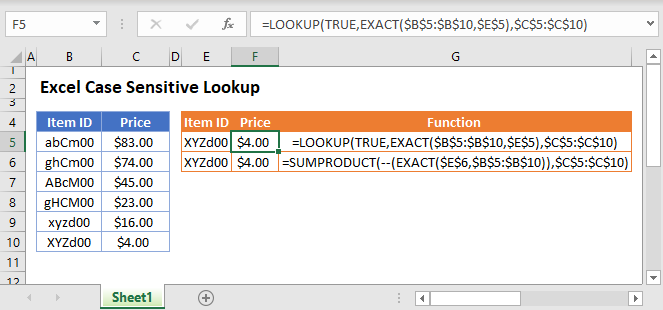## Method 1 – LOOKUP Function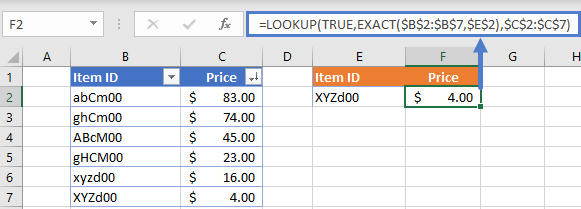### LOOKUP Function

The LOOKUP Function is used to look up an approximate match for a value in a column and returns the corresponding value from another column.

### Case-sensitive Lookup

By combining LOOKUP and EXACT, we can create a case-sensitive lookup formula that returns the corresponding value for our case-sensitive lookup. Let’s walk through an example.

We have a list of items and their corresponding prices (notice that Item ID is case-sensitive unique):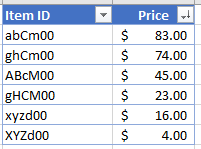Supposed we are asked to price for an item using its Item ID like so: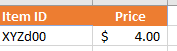To accomplish this, we can use LOOKUP and EXACT in a formula like so:

``=LOOKUP(TRUE,EXACT(<Lookup range>,<lookup value>),<results range>)``
``=LOOKUP(TRUE,EXACT(\$B\$2:\$B\$7,\$E\$2),\$C\$2:\$C\$7)``

Limitation: for this method to work, the values must be sorted in descending order

### How does the formula work?

The EXACT function checks the Item ID in E2 (lookup value) against the values in B2:B7 (lookup range) and returns TRUE where there is an exact match. Then the LOOKUP function returns the corresponding match in C2:C7 (results range) when the nested EXACT returns TRUE.

## Method 2 – SUMPRODUCT Function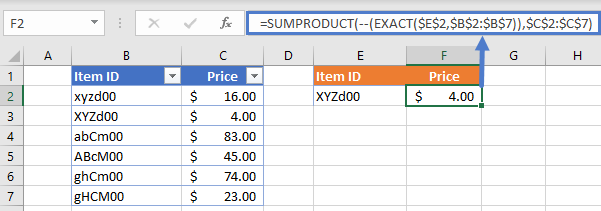### SUMPRODUCT Function

The SUMPRODUCT Function is used to multiply arrays of numbers, summing the resultant array.

### Case-sensitive SUMPRODUCT

Unlike the LOOKUP method, the values do not need to be sorted for this to work. We still need to combine EXACT in a formula to get the results like so:

``=SUMPRODUCT(--(EXACT(<lookup value>,<lookup range>)),<results range>)``
``=SUMPRODUCT(--(EXACT(\$E\$2,\$B\$2:\$B\$7)),\$C\$2:\$C\$7)``

Limitation: The SUMPRODUCT method will only work when the return value (not the lookup value) is numeric.

### How does the formula work?

Like LOOKUP method, the EXACT function deals with finding the case-sensitive match and returns TRUE when there is an exact match or FALSE otherwise. The “–” (known as double unary) converts TRUE to 1 and FALSE to 0. This essentially creates the first array for SUMPRODUCT to multiply with our results array:

``{0,1,0,0,0,0}*{16,4,83,45,74,23} = 4``

## Case Sensitive Lookup in Google Sheets

The Case Sensitive Lookup Function works exactly the same in Google Sheets as in Excel: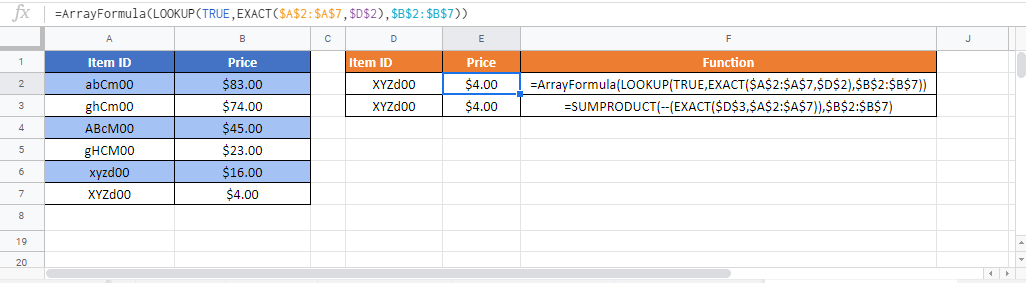### Excel Practice Worksheet

Practice Excel functions and formulas with our 100% free practice worksheets!